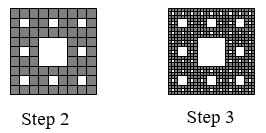$$\newcommand{\id}{\mathrm{id}}$$ $$\newcommand{\Span}{\mathrm{span}}$$ $$\newcommand{\kernel}{\mathrm{null}\,}$$ $$\newcommand{\range}{\mathrm{range}\,}$$ $$\newcommand{\RealPart}{\mathrm{Re}}$$ $$\newcommand{\ImaginaryPart}{\mathrm{Im}}$$ $$\newcommand{\Argument}{\mathrm{Arg}}$$ $$\newcommand{\norm}{\| #1 \|}$$ $$\newcommand{\inner}{\langle #1, #2 \rangle}$$ $$\newcommand{\Span}{\mathrm{span}}$$

# 18.15: Fractals

$$\newcommand{\vecs}{\overset { \rightharpoonup} {\mathbf{#1}} }$$ $$\newcommand{\vecd}{\overset{-\!-\!\rightharpoonup}{\vphantom{a}\smash {#1}}}$$$$\newcommand{\id}{\mathrm{id}}$$ $$\newcommand{\Span}{\mathrm{span}}$$ $$\newcommand{\kernel}{\mathrm{null}\,}$$ $$\newcommand{\range}{\mathrm{range}\,}$$ $$\newcommand{\RealPart}{\mathrm{Re}}$$ $$\newcommand{\ImaginaryPart}{\mathrm{Im}}$$ $$\newcommand{\Argument}{\mathrm{Arg}}$$ $$\newcommand{\norm}{\| #1 \|}$$ $$\newcommand{\inner}{\langle #1, #2 \rangle}$$ $$\newcommand{\Span}{\mathrm{span}}$$ $$\newcommand{\id}{\mathrm{id}}$$ $$\newcommand{\Span}{\mathrm{span}}$$ $$\newcommand{\kernel}{\mathrm{null}\,}$$ $$\newcommand{\range}{\mathrm{range}\,}$$ $$\newcommand{\RealPart}{\mathrm{Re}}$$ $$\newcommand{\ImaginaryPart}{\mathrm{Im}}$$ $$\newcommand{\Argument}{\mathrm{Arg}}$$ $$\newcommand{\norm}{\| #1 \|}$$ $$\newcommand{\inner}{\langle #1, #2 \rangle}$$ $$\newcommand{\Span}{\mathrm{span}}$$

1.3.5.9. Four copies of the Koch curve are needed to create a curve scaled by 3.

$$D=\frac{\log (4)}{\log (3)} \approx 1.262$$11. Eight copies of the shape are needed to make a copy scaled by 3. $$D=\frac{\log (8)}{\log (3)} \approx 1.893$$

13.15. a) $$5-i$$ b) $$5-4 i$$

17. a) $$6+12 i$$ b) $$10-2 i$$ c) $$14+2 i$$

19.$$(2+3 i)(1-i)=5+i$$. It appears that multiplying by $$1-i$$ both scaled the number away from the origin, and rotated it clockwise about 45°.

21.

$$z_{1}=i z_{0}+1=i(2)+1=1+2 i$$

$$z_{2}=i z_{1}+1=i(1+2 i)+1=i-2+1=-1+i$$

$$z_{3}=i z_{2}+1=i(-1+i)+1=-i-1+1=-i$$

23.

$$z_{0}=0$$

$$z_{1}=z_{0}^{2}-0.25=0-0.25=-0.25$$

$$z_{2}=z_{1}^{2}-0.25=(-0.25)^{2}-0.25=-0.1875$$

$$z_{3}=z_{2}^{2}-0.25=(-0.1875)^{2}-0.25=-0.21484$$

$$z_{4}=z_{3}^{2}-0.25=(-0.21484)^{2}-0.25=-0.20384$$

25. attracted, to approximately $$-0.37766+0.14242 i$$

27. periodic 2-cycle 29. Escaping 31. periodic 3-cycle

33. a) Yes, periodic 3-cycle b) Yes, periodic 3-cycle c) No

18.15: Fractals is shared under a CC BY-SA 3.0 license and was authored, remixed, and/or curated by David Lippman via source content that was edited to conform to the style and standards of the LibreTexts platform; a detailed edit history is available upon request.